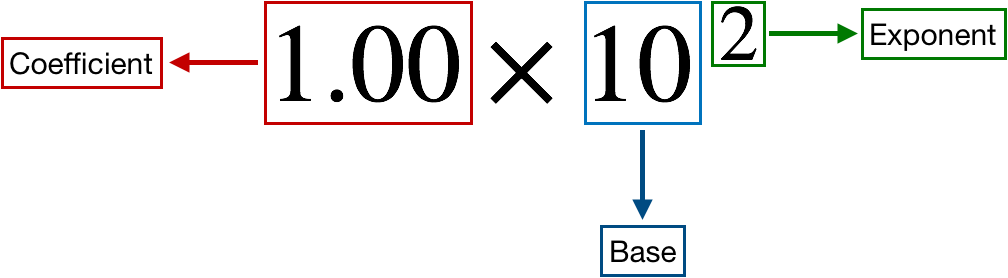# Problem: What is the following number to four significant figures in standard exponential notation: 0.008543210

###### FREE Expert Solution

We’re being asked to express 0.008543210 in scientific notation rounded off to four significant figures.

Recall that a scientific notation expression is composed of three parts:80% (238 ratings)###### Problem Details

What is the following number to four significant figures in standard exponential notation: 0.008543210